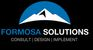# New to Qlik Sense

If you’re new to Qlik Sense, start with this Discussion Board and get up-to-speed quickly.

Announcements
Our next Qlik Insider session will cover new key capabilities. Join us August 11th REGISTER TODAY
cancel
Showing results for
Did you mean:Contributor III

## Sum of aggr function

Hi All,

Im doing a sum of some accounts (P&L accounts) that give me the net sales, the problem i have is that i have to use the "total" because if i dont put it i have zero, also i have to specify the period because if i dont i have the sum of all the periods in the line.

here is the code im using

IF([P&L ACCOUNTS]='TOTAL NET SALES',
Sum(total{<[Period]={'151P'},[P&L ACCOUNTS]={'NET SALES'}>}Amount/1000),

sum(Amount)

)

Labels (2)

• ### Sum if correction needed - HELP

1 Solution

Accepted SolutionsMVP

Try this

``````If([P&L ACCOUNTS] = 'TOTAL NET SALES',
Sum(TOTAL <Period>{<[P&L ACCOUNTS] = {'NET SALES'}>} Amount/1000),
Sum(Amount)
)``````
4 RepliesPartner
Hi,

Can you provide sample data

Regards
FrankMVP

Try this

``````If([P&L ACCOUNTS] = 'TOTAL NET SALES',
Sum(TOTAL <Period>{<[P&L ACCOUNTS] = {'NET SALES'}>} Amount/1000),
Sum(Amount)
)``````Contributor III
Author
for example i have this data
Period Month Country BU Account Currency Amount
151P APR MEX GI Net sales USD 800
151P APR MEX GI OLA Sales USD 0
151P APR MEX GI Comissions USD 0
BUDGET APR MEX GI Net sales USD 100
BUDGET APR MEX GI OLA Sales USD 0
BUDGET APR MEX GI Comissions USD 0

my net sales is 'Net sales' + 'OLA sales' + 'Comissions' and they are under the dimension = 'NET SALES'

when i do the sum of
Sum(total{<[[P&L ACCOUNTS]={'NET SALES'}>}Amount/1000) in the period of budget and 151p i have the same number of 900 but if i remove the total i got zero in both cases

the result has to be 800 in 151P and 100 in budgetContributor III
Author

Thank you!!! it worksTags
Community Browser